# Enhanced Equal Frequency Partition Method for the Identification

• Slides: 14
Download presentation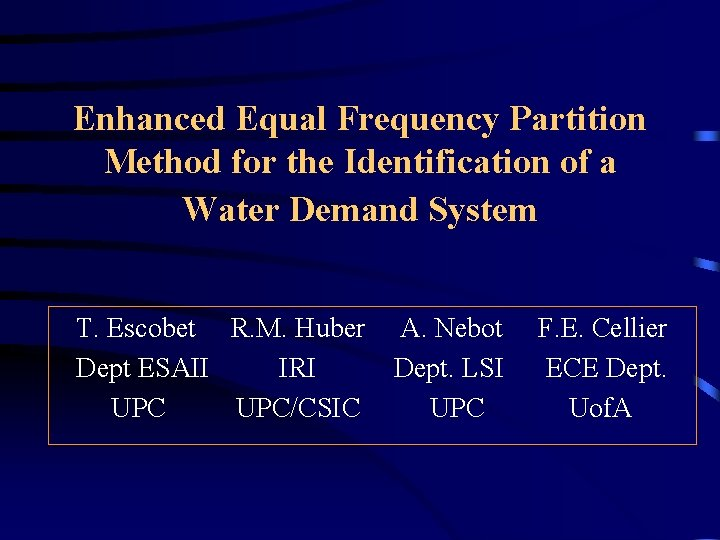Enhanced Equal Frequency Partition Method for the Identification of a Water Demand System T. Escobet R. M. Huber Dept ESAII IRI UPC/CSIC A. Nebot Dept. LSI UPC F. E. Cellier ECE Dept. Uof. A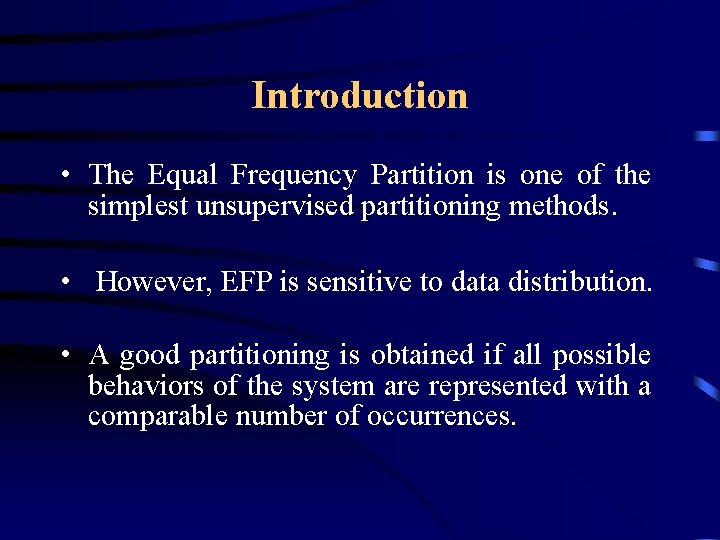Introduction • The Equal Frequency Partition is one of the simplest unsupervised partitioning methods. • However, EFP is sensitive to data distribution. • A good partitioning is obtained if all possible behaviors of the system are represented with a comparable number of occurrences.Introduction • The first goal is to present an enhancement to the EFP method to be used within the FIR methodology that allows to reduce, to some extent, the data distribution dependency. • The second goal is to use the EEFP method within the discretization step of FIR for the identification of a model of a water demand system.Enhanced EFP method ¬The EEFP method eliminates multiple observations of the same behavioral pattern. δ = range of similar observations. α = minimum number of occurrences to assume that this behavioral pattern is over-represented.FIR fuzzification process Then applies EFP to the remaining set of significantly different patterns to decide on a meaningful set of landmarks.Water demand application • The system to be modeled is the water distribution network of the city of Sintra in Portugal.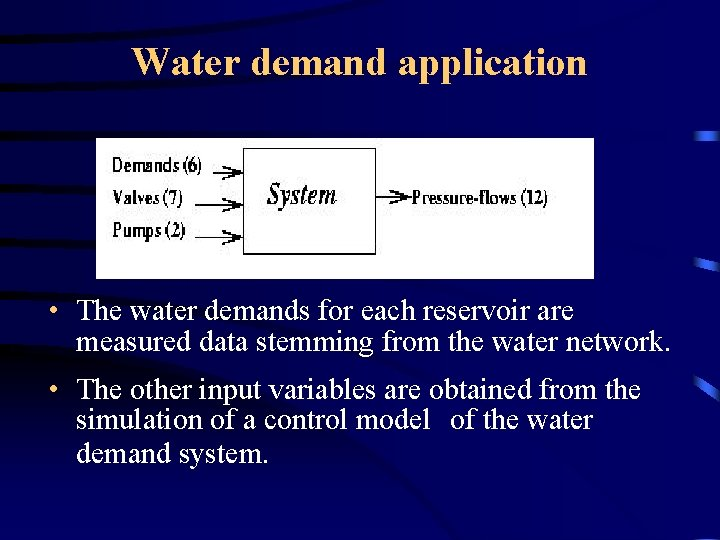Water demand application • The water demands for each reservoir are measured data stemming from the water network. • The other input variables are obtained from the simulation of a control model of the water demand system.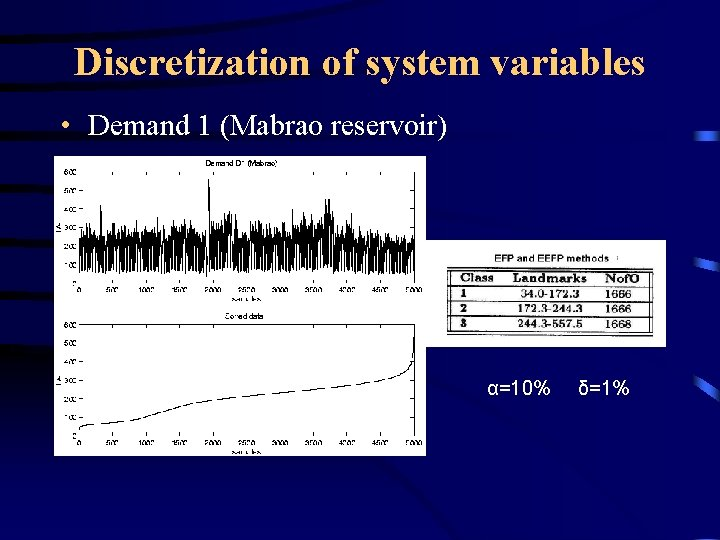Discretization of system variables • Demand 1 (Mabrao reservoir) α=10% δ=1%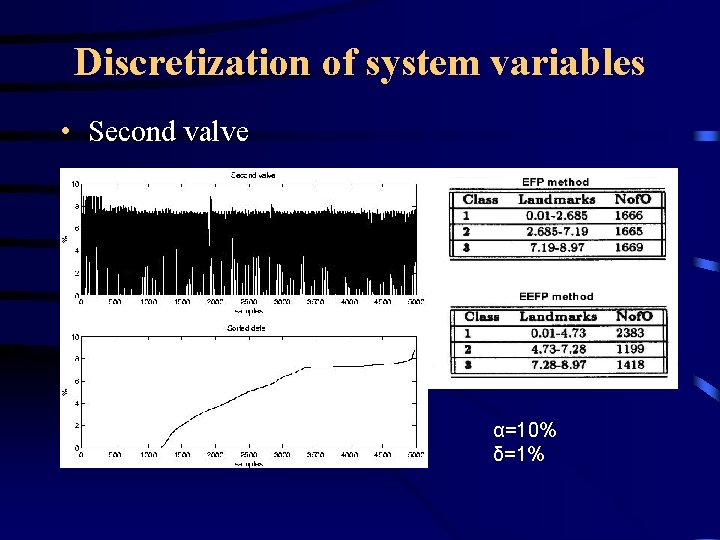Discretization of system variables • Second valve α=10% δ=1%Discretization of system variables • The last input variable is the state of the pumps. • Each pump is composed of two motors, that can either be both stopped, both pumping, or one stopped and one pumping.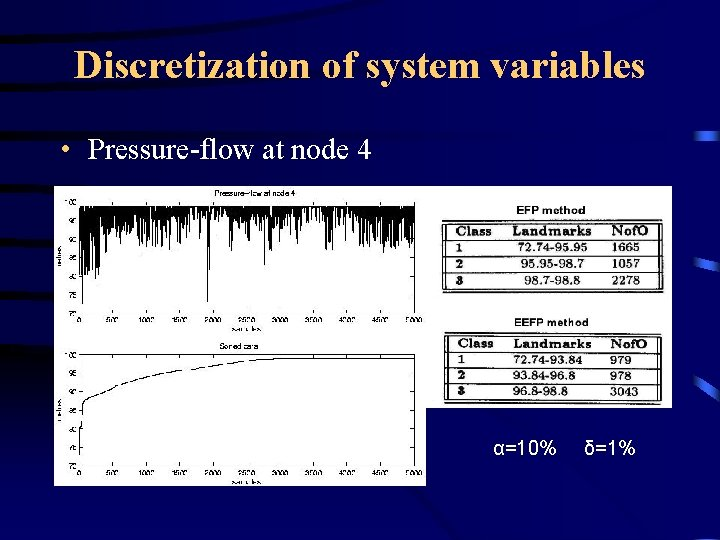Discretization of system variables • Pressure-flow at node 4 α=10% δ=1%Pressure-flow models errorsPrediction of the pressure-flow at node 4 FIR prediction with EFP (upper) and EEFP (lower)Conclusions • In this paper an enhancement to the classical Equal Frequency Partition method is presented. • The EEFP method allows to obtain a better distribution of the data into classes. • A real application i. e. water distribution network is studied. • The prediction errors obtained when the EEFP method is used in the fuzzification process are lower than the ones obtained when the classical EFP method is used.